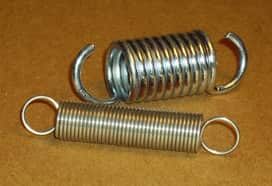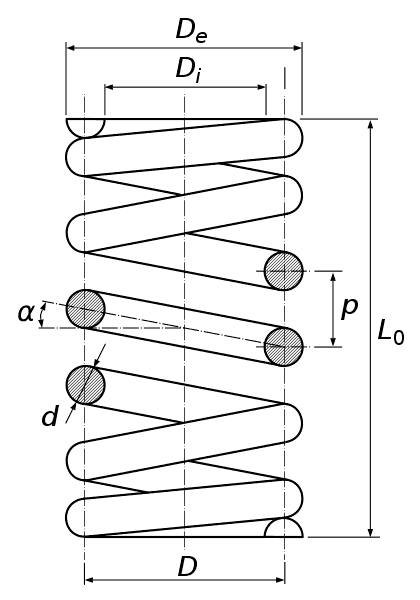# Helical springs - its types, terminology, and advantages with PDF

Today at The Mechanical post you would be reading about What are Helical springs? its types, advantages, and terminology related to it.

You can get your copy of the PDF on Helical springs at the end of this article!

## What is a spring?

Spring can be defined as an elastic body that resists an externally applied force by causing a change in its shape and size and when the load is removed the spring regains its original shape and size.

Springs work on the principle of Hooke's law which states that stress applied to the spring is directly proportional to the strain produced within the elastic limit.

In simpler words, the extension of the spring is directly proportional to the stress applied within the elastic limit. So the greater the stress applied, the greater will be the deflection produced.

We have a detailed article in simple words on Hooke's law so don't forget to check it out.

Now that we know what is a spring let's get acquainted with helical springs

## What is a helical spring?

As the name suggests, a helical spring is a spring made up of a wire wound in the shape of a helix. The spring wire cross-section may be either of circular, rectangular, or square shape.

### Classification of Helical spring

The following is the classification of helical springs.

#### Based on nature of the load

• Helical tension spring
• Helical compression spring
• Helical torsion spring

#### Based on the helix angle

• Open coil helical spring
• Closed coil helical spring

Let us now see each of them in brief.

#### Helical tension spring

The helical tension spring is designed to withstand the axial tensile load. The coils are very close to each other as shown in the figure. When a tensile load is applied axially, the spring tends to expand and the distance between the coils increases.When tension is applied the spring resists pulling and tries to hold itself together.

Applications of helical tension spring include springs in a trampoline, carburetors, door locks, dial indicator, gym equipment, Parkinson's gear tester, etc.

#### Helical compression spring

The helical compression spring is a helical spring wherein the coils are apart from each other and as the name suggests, the spring is designed to withstand the axial compressive load.

When compressive forces act on it, the spring coils compress and come close to each other. If the load is removed, the spring returns back to its original shape.

Applications of helical compression spring include shock absorbers in automobiles, suspension in cycles, applying pressure in a clutch of an automobile, in brakes of automobiles, in spring balances for measurement of force, toys, etc.

#### Helical torsion spring

As the name suggests, in the helical torsion spring the force is applied tangentially to the axis and the spring is subjected to twisting by the forces acting on it.

When the spring is subjected to twisting, it exerts torque in the opposite direction and is proportional to the amount angle it is being twisted.

Applications of Helical torsion spring include as simple as the spring in hair clips, mouse traps, hand gripper in gyms, robotics, and many more

#### Open coil Helical spring

An open coil helical spring is a spring that has a helix angle greater than 10 degrees. There is a considerable amount of gap between two adjacent coils of the spring.

#### Closed coil Helical springImage Source: http://en.wikipedia.org/wiki/Image:Springs_009.jpg

In a closed coil helical spring, the helix angle is less than 10 degrees. The gap between the two adjacent coils is comparatively small.

### Helical spring terminology

The following are the terms used for helical spring.

#### 1. Solid length

When all the coils of the spring are compressed such that they come in contact with each other then the length of the spring is said to be the solid length.

Mathematically,  Solid length = Total no. of coils × Spring wire diameter (d)Image source - Yapparina, CC0, via Wikimedia Commons

#### 2. Free length (L0)

The free length of the spring is the length of the spring in an unloaded or free condition.

#### 3. Mean diameter (D)

The mean diameter of the spring is the average of the outer coil diameter (De) and the inner coil diameter (D).

Mathematically, Mean diameter = (De + D) / 2

#### 4. Pitch (P)

The pitch of the spring is defined as the axial distance between two adjacent coils in unloaded condition.

#### 5. Spring Index (C)

Spring index can be defined as the ratio of the mean diameter of spring (D) to the spring wire diameter (d)

Mathematically we can say, Spring Index (C) = D / d

#### 6. Helix angle of spring (α)

The helix angle of the spring is the angle made by the spring wire axis and a line perpendicular to the axis of the spring as shown in the above figure.

### Advantages of Helical springs

The following are the advantages of helical springs
• Helical springs are highly reliable.
• They have a constant spring rate.
• Helical springs possess good durability.
• Helical springs are available in a wide range according to the application.

𑁋𑁋𑁋𑁋𑁋𑁋𑁋𑁋𑁋𑁋𑁋𑁋𑁋𑁋

So That's a brief on Helical springs, we'll be back with another interesting article!

Also, do let us know in the comments about your thoughts as well as spread the word among the fellow mechanical engineers with the social links given below.

Till then keep learning and reading The Mechanical post!